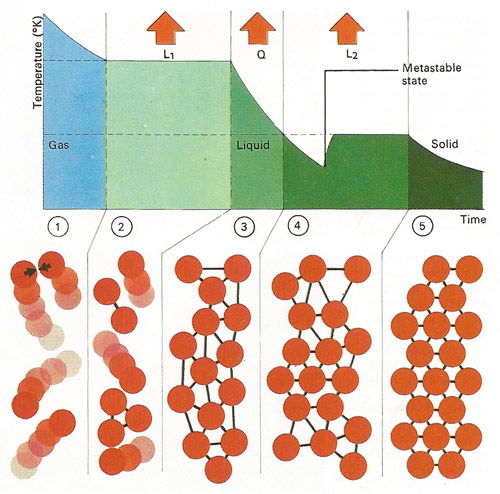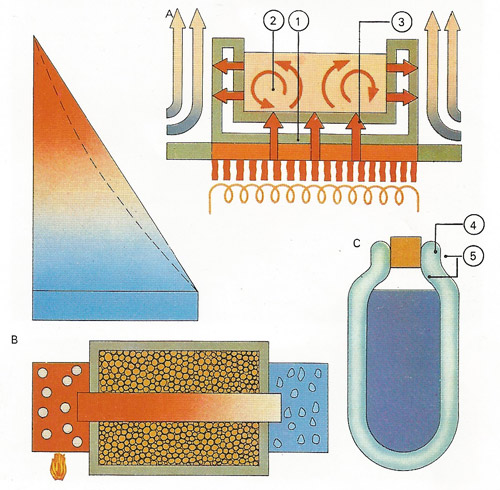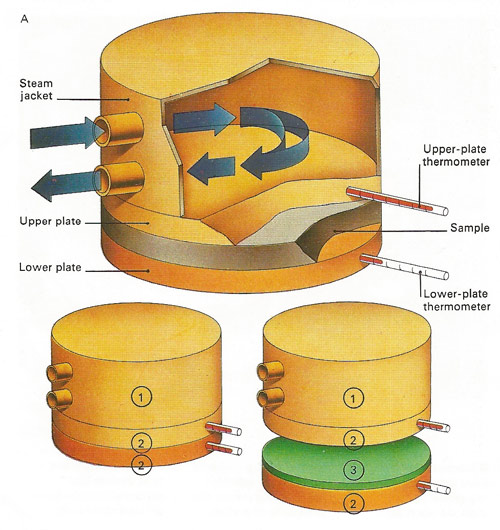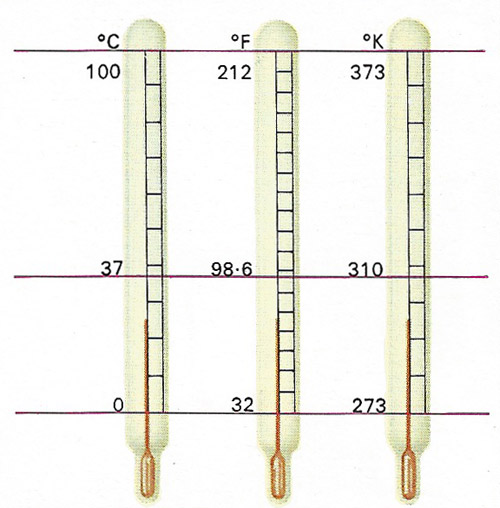A# heatVibration of atoms and molecules in a substance governs its temperature. In a gas  atoms move independently and their average velocity and mass determine the internal energy and temperature. After cooling, loss of latent heat of condensation [L1] converts the gas at boiling-point to a liquid state  when its atoms become locked in a weakly bonded arrangement. Further cooling  to freezing point loses a quantity of heat [Q] and a solid then forms through  release of latent heat of fusion [L2]; the atoms then become rigidly bonded together . A colder "metastable" phase can precede actual freezing.The three ways in which heat moves all take place when a pan is heated [A] – conduction through the metal walls of the pan , convection by fluid motion  and radiation from the heat source to the pan . In theory an insulated good conductor with ice at one end and boiling water at the other varies in temperature linearly with distance along the bar [B], as in the straight-line graph. With poor insulation a curve like the dotted line results. A vacuum flask [C] has a vacuum  to prevent conduction and convection and silvered walls , to minimize heat loss by radiation.Conductivity is the amount of heat passing in unit time across unit cross-section per unit of temperature gradient. It can be measured [see A, above] by noting the time taken for a known quantity of heat to pass through the sample. Two plates of equal area  are put against the material . Thermometers on each side measure the temperatures as the upper plate is heated by a steam jacket .Materials of high conductivity are tested in a cylinder  heated at one end by steam  to 100°C . Other thermometers  measure the temperature of the sample and the rise in temperature of water circulating through a jacket attached to the other end , and give a way of calculating conductivity.Temperature scales are arbitrary both in their range and in their division into degrees. The freezing-point of water is set at 32 Fahrenheit (F), 0 Celsius or centigrade (C) and 273 Kelvin (K). Between this point and the boiling-point of water there are 180°F, 100°C and 100K. The Kelvin scale is unique in setting its lowest fixed point at absolute zero, which in practice is unobtainable.The indicators in a maximum and minimum thermometer [A] are pushed along by mercury in a U-tube and stay put at the furthest point of travel. Liquid in a cylindrical reservoir senses the temperature and its contraction [B] or expansion [C] displaces the mercury along scales at each side of the U. The steel indicators can be reset simply by moving a small magnet along the outside of the tube.A bimetallic strip thermometer uses a helical metal strip that unwinds when heated and rotates a pointer over a calibrated scale. When warmed, the inner metal (usually copper) expands more than the outer one, so causing the bimetallic strip to unwind. The material used for the outer strip is usually invar, and alloy of iron and nickel, which has a low coefficient of thermal expansion.

Heat is a form of energy associated with the motion (translational, rotational, or vibrational) of atoms and molecules. It is transferred as a result of a temperature difference, and is conducted through solid and fluid media by conduction, through fluid media by convection, and through empty space by radiation.

The heat contained by a body is the product of its mass, temperature, and specific heat, and is measured in calories or joules. (An older unit, the British thermal unit, or Btu, is still sometimes used in engineering applications, especially in the US.)

The chief observable physical effects of a change in the heat content of a body may include rise in temperature; change of state from solid to liquid (melting), solid to gas (sublimation), and liquid to gas (evaporation); expansion; and electrical effects such as the Peltier effect and Seebeck effect.

## Development of ideas about heat

The scientific concept of heat is derived from our sense-perception, and the thermometer enables us to define a scale with which to measure its "intensity." Galileo invented the first thermometer and Amontons first used mercury. Different scales were introduced by Fahrenheit, Réamur, and Celsius.

The caloric theory of heat was a view, formalized by Antoine Lavoisier toward the end of the eighteenth century, that heat consists of particles of a weightless, invisible fluid, caloric, which resides between the atoms of material substances. The theory fell from favor as physicists began to appreciate the equivalence of work and heat.

The idea of heat as a quantity was suggested by observation in distilleries, but it was Joseph Black who cleared up the still existing confusion between heat and temperature, calling them quantity and intensity of heat. He studied the change of state from ice to water and water to steam, finding that, in each case, much heat was absorbed with no rise in temperature; heat, as he said, was rendered latent (see latent heat). He explained the different amounts of heat needed by different substances to produce the same rise in temperature by assigning to each substance a "specific heat," and showed how to measure quantities of heat in a calorimeter – a vessel containing a known mass of weight with a thermometer immersed in it.

## Towards a new theory of heat

It is evident that these experiments needed a theory of heat laying stress on the idea of a quantity which remained constant as the heat passed from one body to another. Although Henry Cavendish, Robert Boyle, and Isaac Newton had regarded heat as a vibratory motion of the particles of substances, that view did not lend itself, before the days of the theory of energy, to the idea of a quantity remaining constant. It was better to take the alternative hypothesis that heat was a subtle, invisible, weightless fluid, passing freely between the particles of bodies, and this caloric theory served well until the middle of the nineteenth century.

The calorists explained the heat developed by friction by supposing that the filings or abrasions, or the main body after friction, had a lower specific heat, so that heat was, as it were, squeezed out. Though the American Benjamin Thompson, who in Bavaria became famous as Count Rumford, had shown in 1798 by experiments on the boring of cannon that the heat developed was proportional to the work done, and had no relation to the amount of shavings, his work was forgotten or ignored, and the caloric theory flourished.

But by 1840 it had become apparent that some of the powers of nature were mutually convertible. Assuming that when air is compressed all the work appears as heat, J. R. Mayer calculated the mechanical equivalent of a unit of heat. W. R. Grove wrote on the "Correlation of Physical Forces," and Hermann von Helmholtz published Ueber die Erhaltung der Kraft. The idea of correlation was in the air.

## Mechanical equivalent of heat

From 1840 to 1850 James Joule was engaged in measuring experimentally the heat liberated by mechanical and electrical work. He found that, however the work was done, the expenditure of the same amount of work produced the same quantity of heat. To warm one pound of water through one degree Fahrenheit needed about 772 foot pounds of work – a figure afterwards corrected to 778. This became known as the mechanical equivalent of heat.

## Heat as a form of energy

A troublesome double meaning in the word "force," which had been pointed out by Thomas Young, was cleared up when Rankine and William Thomson (Lord Kelvin) used the word "energy" in a specialized sense to denote the power of doing work, and measured, if the transformation is complete, by the work done. Joule's experiments showed that the total amount of energy in an isolated system is constant, the quantity lost in work reappearing as heat. Thus, the somewhat vague "correlation of forces" became the quite definite "conservation of energy." And the law of conservation of energy, in turn, became the first law of thermodynamics.

## Birth of statistical mechanics

If heat is a form of energy, it must consist in motion of molecules. This kinetic theory, given in early forms by Bernoulli, Waterston, and Joule, was first properly expounded by Rudolf Clausius in 1857. The chances of molecular collision will produce molecules moving in all directions with all velocities, the average value of which was calculated by Joule; for hydrogen it is 1,844 meters (more than a mile) per second, and for oxygen 461 meters per second. But James Clerk Maxwell and Ludwig Boltzmann applied to the velocities a law of error derived by Laplace and by Gauss from Pascal's theory of probability. Maxwell and Boltzmann also showed that, on the kinetic theory, the total energy of a molecule should be divided equally among its degrees of freedom, that is the number of coordinates needed to specify its position and condition. From this it follows that the ration of the specific heats of a gas at constant pressure and at constant volume is 1.67 for three degrees of freedom and 1.4 for five – figures confirmed experimentally for gases with monatomic and diatomic molecules respectively.

## Joule heat

Joule heat is the heat produced because of the passage of an electrical current. The Joule heat is given by I 2R, where I is the current and R the resistance.

## Thermal agitation and molecular motion

A bicycle becomes warm when pumping up a tire, an effect that can be explained by the kinetic theory of heat, first suspected by Isaac Newton and developed by eighteenth and nineteenth century scientists. The theory describes heat as the kinetic energy (energy of motion) of the vibrating atoms or molecules that make up every substance. In the bicycle pump, air molecules are speeded up by collisions with the pump's piston. The increase in their kinetic energy takes the form of heat.

According to the third law of thermodynamics, absolute zero – the temperature (-273.16°C) at which molecules cease all motion – is unobtainable. As a result, molecules perform a continuous motion known as "thermal agitation", which increases in vigor as heat is transferred to an object. Indirect evidence of this incessant motion was first obtained by the botanist Robert Brown in 1827. He discovered that tiny pollen grains suspended in water were continually making jerky movements. It is the continual unequal bombardment of each tiny speck by the molecules of the liquid that produces this "Brownian motion". The smaller the particle, the more violent is the motion.

The kinetic theory also explains why, when a hot gas is mixed with a cooler one, a common temperature is eventually reached. Molecular kinetic energy of the hot gas molecules is transferred by thousands of collisions to the cold gas molecules, until the average kinetic energies of both gases are the same. The molecules all travel with different velocities, changing after each collision. For this reason the temperature of the gas (or other substance) is a measure of the average molecular kinetic energy.

The changes of state that may occur when a substance is heated can be described by the kinetic theory. In a solid, the atoms or molecules are tightly bound together and vibrate only about an average position. As the solid is heated its internal kinetic energy increases and the particles vibrate more vigorously. They move farther and farther apart until eventually the attraction between them is insufficient to keep them in a fixed position. They can then slide about and exchange partners – the solid melts and a liquid is formed. The heat needed to achieve this change of state from tightly bound solid to loosely bound liquid at the same temperature is called the latent heat of fusion. If yet more heat is applied, the atoms or molecules gain more kinetic energy, move with greater velocity within the liquid and the proportion of these escaping from the surface increases (vapor pressure increases). Eventually, at the boiling-point, so many atoms have enough energy to escape from the liquid that the vapor pressure equals atmospheric pressure. In the gaseous state the atoms or molecules move almost independently; the conversion of a liquid at its boiling-point into a gas requires latent heat of vaporization.

## Changes of temperature

Instead of changing the state of a substance (for which latent heat is needed), applied heat may merely raise its temperature. The temperature change depends directly on the quantity of heat transferred and this is measured in units called calories or joules (4.2 joules = 1 calorie). The calorie is defined as the quantity of heat that raises the temperature of 1 gram of water by 1°C. So the quantity of heat needed to raise the temperature of 1 gramme of any substance by 1°C can be measured in these units – and this is called the specific heat of the substance. The quantity of heat that raises the whole bulk of substance by 1°C is called the thermal capacity of the given mass.

It is possible for heat to be transferred from place to place and there are three ways in which this can occur: by conduction, convection and radiation. The first two methods rely on the fact that atoms that have received kinetic energy from a heat source can, in collision, transmit this to their neighbors. In a tightly bound solid only nearest-neighbor collisions occur and any heat transfer through the solid is called conduction. In a fluid (liquid or gas), the medium itself can move and transport atoms of high kinetic energy to the cooler parts of the fluid where they then transfer their heat this is convection. Even when no physical contact exists between atoms, heat can still be transferred. For instance, heat from the Sun reaches the Earth through the near vacuum of space. This method is radiation. Different substances conduct heat at different rates. Their ability to transmit heat is known as their thermal conductivity.

## Measuring temperature

All methods of measuring temperature changes are based on the ways in which materials change physically when heated. The most commonly used characteristic is the expansion of solids or liquids when heated. Usually the physical change caused by a temperature change is made visible against a calibrated scale on the measuring instrument or thermometer. In making any type of thermometer, two constant temperatures or "fixed points" must first be marked. The range between them can then be subdivided as finely as desired. The numbers assigned to the fixed points and the number of degrees between them define the temperature scale, such as Celsius (or centigrade), Fahrenheit and Kelvin (or absolute).